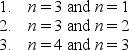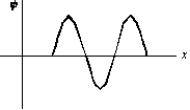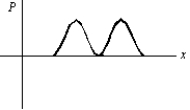# Fundamentals of Physics Study Set 2

Physics & Astronomy

## Quiz 39 :More About Matter WavesStudy FlashcardsLooking for Introductory Physics Homework Help?## Quiz 39 :More About Matter Waves

Showing 1 - 20 of 41An electron confined in a one-dimensional infinite potential well has an energy of 180 eV.What is its wavelength?
Free
Multiple Choice

BThe ground state energy of an electron in a one-dimensional trap with zero potential energy in the interior and infinite potential energy at the walls is 2.0 eV.If the width of the well is doubled, the ground state energy will be:
Free
Multiple Choice

AAn electron is in a one-dimensional trap with zero potential energy in the interior and infinite potential energy at the walls.The ratio E3/E1 of the energy for n = 3 to that for n = 1 is:
Free
Multiple Choice

DIdentical particles are trapped in one-dimensional wells with infinite potential energy at the walls.The widths L of the traps and the quantum numbers n of the particles areRank them according to the kinetic energies of the particles, least to greatest.
Multiple ChoiceFour different particles are trapped in one-dimensional wells with infinite potential energy at their walls.The masses of the particles and the width of the wells areRank them according to the kinetic energies of the particles when they are in their ground states, lowest to highest.
Multiple ChoiceThe ground state energy of an electron in a one-dimensional trap with zero potential energy in the interior and infinite potential energy at the walls:
Multiple ChoiceTwo one-dimensional traps have infinite potential energy at their walls.Trap A has width L and trap B has width 2L.For which value of the quantum number n does a particle in trap B have the same energy as a particle in the ground state of trap A?
Multiple ChoiceAn electron is trapped in a deep well with a width of 0.3 nm.If it is in the state with quantum number n = 3 its kinetic energy is:
Multiple ChoiceA particle is trapped in a one-dimensional well with infinite potential energy at the walls.Three possible pairs of energy levels areOrder these pairs according to the difference in energy, least to greatest.
Multiple ChoiceAn electron in an atom initially has an energy 5.5 eV above the ground state energy.It drops to a state with energy 3.2 eV above the ground state energy and emits a photon in the process.The wave associated with the photon has a wavelength of:
Multiple ChoiceAn electron in an atom drops from an energy level at -1.1 10-18 J to an energy level at -2.4 10-18 J.The wave associated with the emitted photon has a frequency of:
Multiple ChoiceAn electron in an atom initially has an energy 7.5 eV above the ground state energy.It drops to a state with an energy of 3.2 eV above the ground state energy and emits a photon in the process.The momentum of the photon is:
Multiple ChoiceAn electron is in a one-dimensional trap with zero potential energy in the interior and infinite potential energy at the walls.A graph of its wave function (x)versus x is shown.The value of quantum number n is:Multiple ChoiceAn electron is in a one-dimensional trap with zero potential energy in the interior and infinite potential energy at the walls.A graph of its probability density P(x)versus x is shown.The value of the quantum number n is:Multiple ChoiceA particle is trapped in an infinite potential energy well.It is in the state with quantum number n = 14.How many nodes does the probability density have (counting the nodes at the ends of the well)?
Multiple ChoiceA particle is trapped in an infinite potential energy well.It is in the state with quantum number n = 14.How many maxima does the probability density have?
Multiple ChoiceA particle is confined to a one-dimensional trap by infinite potential energy walls.Of the following states, designed by the quantum number n, for which one is the probability density greatest near the center of the well?
Multiple ChoiceIf a wave function for a particle moving along the x axis is "normalized" then:
Multiple Choice